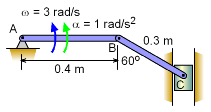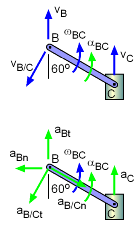Ch 5. Rigid Body General Motion Multimedia Engineering Dynamics Fixed Axis Rotation Plane Motion Velocities Zero Velocity Point Plane Motion Accelerations Multiple Gears Rot. Coord. Velocities Rot. Coord. Acceleration
 Chapter - Particle - 1. General Motion 2. Force & Accel. 3. Energy 4. Momentum - Rigid Body - 5. General Motion 6. Force & Accel. 7. Energy 8. Momentum 9. 3-D Motion 10. Vibrations Appendix Basic Math Units Basic Equations Sections Search eBooks Dynamics Fluids Math Mechanics Statics Thermodynamics Author(s): Kurt Gramoll ©Kurt GramollDYNAMICS - EXAMPLESliding Piston Example Two bars link a vertically sliding piston, C, to a pinned point, A. If bar AB rotates at a rate of 3 rad/s and accelerates at a rate of 1 m/s2, what is the angular acceleration of bar BC? SolutionVelocity and Acceleration Diagrams Velocity information about bar BC must first be found before accelerations can be determined. The key object in this system is rod BC. Velocity of points B can be found, and velocity direction of C can be found. Velocity First, find the velocity of point B by relating its velocity to fixed point A,      vB = vA + vB/A = 0 + ωAB × rAB           = 3k × 0.40i = 1.2j m/s The velocity direction of C is known, thus      vc = vcj m/s Combining gives,      vB = vC + vB/C = vC + ωBC× rBC      1.2j = vCj + ωBCk × (-0.3 sin60i + 0.3 cos60j)      1.2j = vCj + 0.2598ωBCj + 0.15ωBCi      i terms:  ωBC = 0 Acceleration Now the acceleration terms can be determined since the velocity information has been calculated. The acceleration of point B is,      aB = aA + aA/B          = aA + ωAB2 rAB + αAB × rAB          = 0 + (3)2 (0.4i) +1k × 0.4i          = 3.6i + 0.4j Acceleration of point C,      aC = acj m/s2 Combining,      aB = aC + aB/C      aB = aC + ωBC2 rBC + αBC × rBC      3.6i + 0.4j = aCj + 0 +                   + aBCk × (-0.3 sin60i + 0.3 cos60j)      3.6i + 0.4j = aCj + 0.2598 aBCj + 0.15αBCi      i terms:   -3.6 = -0.15 αBC αBC = 24 rad/s2

Practice Homework and Test problems now available in the 'Eng Dynamics' mobile app
Includes over 400 problems with complete detailed solutions.
Available now at the Google Play Store and Apple App Store.